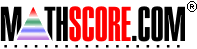Math Practice Online > free > lessons > Florida > 6th grade > Fraction Word Problems 2

## Fraction Word Problems 2

Solving word problems that involve fraction multiplication and division. You may need pencil and paper.

 Sample Problems for Fraction Word Problems 2 Lesson for Fraction Word Problems 2

### This topic aligns to the following state standards

Grade 5: Num 1. Solves real-world problems involving addition, subtraction, multiplication, and division of whole numbers, and addition, subtraction, and multiplication of decimals, fractions, and mixed numbers using an appropriate method (for example, mental math, pencil and paper, calculator).
Grade 6: Num 1. Knows the appropriate operations to solve real-world problems involving whole numbers, decimals, and fractions.
Grade 6: Num 2. Solves real-world problems involving whole numbers, fractions, decimals, and common percents using one or two-step problems.
Grade 7: Num 1. Knows the appropriate operation to solve real-world problems involving fractions, decimals, and integers.
Grade 7: Num 1. Solves multi-step real-world problems involving whole numbers, fractions or decimals using appropriate methods of computation, such as mental computation, paper and pencil, and calculator.
Grade 8: Num 1. Solves multi-step real-world problems involving fractions, decimals, and integers using appropriate methods of computation, such as mental computation, paper and pencil, and calculator.

Copyright Accurate Learning Systems Corporation 2008.